Name:    Physics-Math/Motion Unit 1 Test

Matching

Match each item with the correct statement below concerning STANDARD SI units.
 a. kelvin f. kilometer b. celsius g. minute c. gram h. second d. kilogram i. watt e. meter j. joule

1.

SI unit of time

2.

SI unit of length

3.

SI unit of temperature

4.

SI unit of mass

5.

SI unit for energy

True/False
Indicate whether the statement is true or false.

6.

Exponents must match when adding or subtracting numbers in scientific notion.

7.

In most line graphs, the dependent variable is placed along the X-axis.

8.

If you were trying to get out of the way of a storm, you would need to know the speed at which it was moving.

9.

Acceleration occurs when velocity changes.

10.

If one toy car is traveling at 10 cm/s and another toy car is moving at 10 cm/s in the opposite direction, both cars have the same velocity.

11.

A straight line on an acceleration graph indicates constant speed.

12.

A straight, slanted line on a graph of distance versus time means that the object has zero acceleration..

13.

A single point on a speed graph give instantaneous speed.

Multiple Choice
Identify the choice that best completes the statement or answers the question.

14.

How is 0.00099 written in scientific notation?
 a. 99 ´ 10–5 b. 9.9 ´ 10–4 c. 0.99 ´ 10–3 d. 9.9 ´ 104

15.

There are 1660 megawatts of wind-generated electricity produced globally every year. This amount is equivalent to
 a. 1,660,000 watts c. 16,600,000 watts b. 1,660,000 kilowatts d. 166,000 kilowatts

16.

The diameter of a carbon atom is 0.000 000 000 154 m. What is this number expressed in scientific notation?
 a. 1.54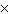10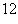m c. 1.5410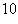m b. 1.5410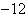m d. 1.5410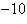m

17.

What is the quantity 0.0075 meters expressed in centimeters?
 a. 0.075 cm b. 0.75 cm c. 7.5 cm d. 70.5 cm

18.

The expression of 3585 km in scientific notation is ____.
 a. 35.8510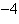km c. 3.58510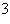km b. 3.58510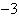km d. 3.58510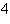km

19.

THIS PROBLEM IS FIXED NOW!
MULTIPLY  4.010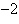m and 8.010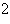m using PROPER scientific notation.
 a. 0.32100 b. 0.32 c. 3.210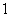d. 3.210-1

20.

How many meters are there in 1,865 cm?
 a. 0.1865 b. 1.865 c. 18.65 d. 186.5

21.

The symbol mm represents a
 a. micrometer. b. millimeter. c. megameter. d. manometer.

22.

The radius of Earth is 6 370 000 m. Express this measurement in km in scientific notation.
 a.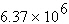km c.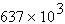km b.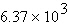km d.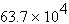km

23.

The Greek letter D (delta) indicates a(n)
 a. difference or change. c. direct proportion. b. sum or total. d. inverse proportion.

24.

If you ride your bicycle down a straight road for 500 m then turn around and ride back, your distance is ____ your displacement.
 a. greater than c. less than b. equal to d. can’t determine

25.

The speed you read on a speedometer is ____.
 a. instantaneous speed c. average speed b. constant speed d. velocity

26.

3 m/s north is an example of a(n) ____.
 a. speed b. velocity c. position d. acceleration

27.

A merry-go-round horse moves at a constant speed but at a changing ____.
 a. velocity c. inertia b. dimension d. none of the above.

28.

Acceleration is rate of change of ____.
 a. position b. time c. velocity d. force

29.

A horizontal line on a velocity/time graph shows ____ acceleration.
 a. positive b. negative c. changing d. zero

30.

What is the speed of an object at rest?
 a. 0.0 m/s b. 1.0 m/s c. 9.8 m/s d. 9.81 m/s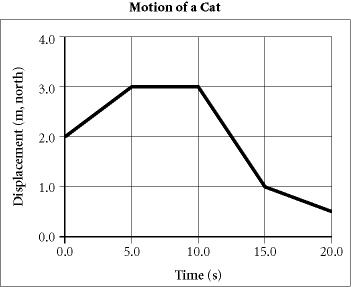31.

According to the graph above, during which interval is the cat at rest?
 a. 0.0–5.0 s c. 10.0–15.0 s b. 5.0–10.0 s d. 15.0–20.0 s

32.

According to the graph above, the cat has a negative slope during which interval?
 a. 0.0–5.0 s c. 10.0–15.0 s b. 5.0–10.0 s d. 10.0–20.0 s

33.

According to the graph above, during which interval does the cat have the greatest velocity?
 a. 0.0–5.0 s c. 10.0–15.0 s b. 5.0–10.0 s d. 15.0–20.0 s

34.

Which of the following line segments on a position versus time graph is physically impossible?
 a. a horizontal line c. a straight line that slopes to the right b. a straight line that slopes to the left d. a vertical line

35.

A train that travels 100 kilometers in 4 hours is traveling at what average speed?
 a. 50 km/h b. 100 km/h c. 2 km/h d. 25 km/h

36.

In graphing motion, the steepness of the slope depends on
 a. how quickly or slowly the object is moving. b. how far the object has moved. c. when the object began moving. d. the direction the object is moving.